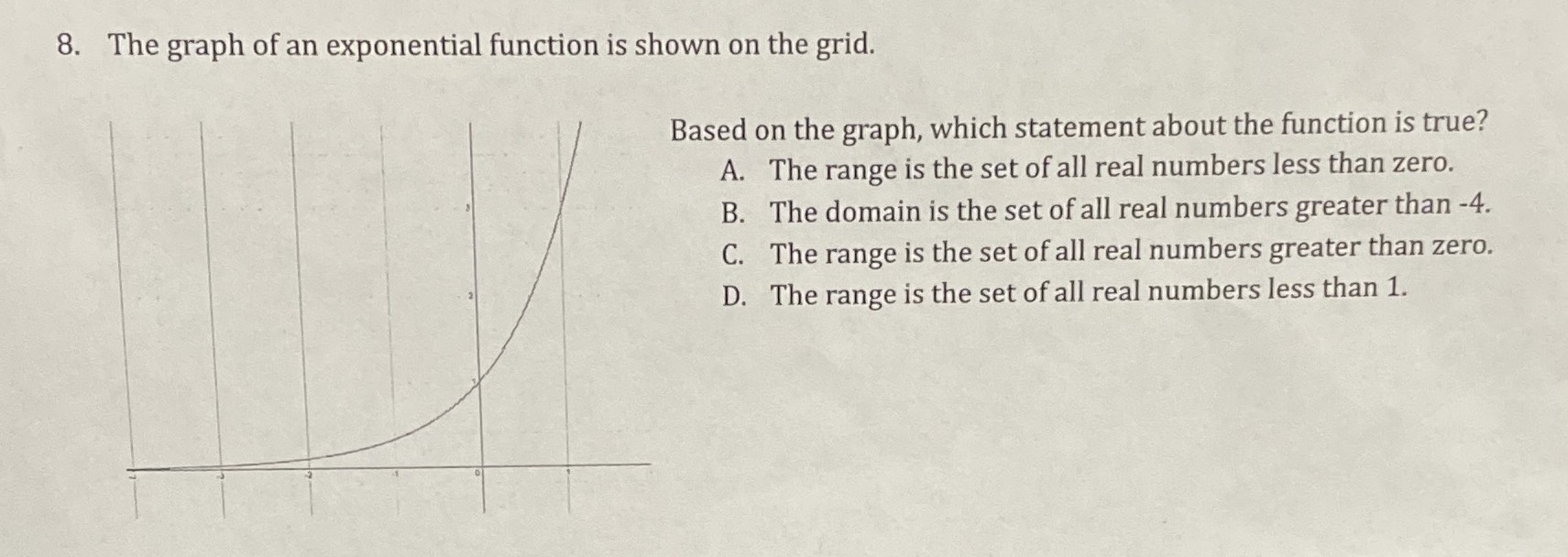### ¿Todavía tienes preguntas de matemáticas?

Pregunte a nuestros tutores expertos
Algebra
Pregunta8. The graph of an exponential function is shown on the grid.

Based on the graph, which statement about the function is true?

A. The range is the set of all real numbers less than zero.

B. The domain is the set of all real numbers greater than $$- 4$$ .

C. The range is the set of all real numbers greater than zero.

D. The range is the set of all real numbers less than $$1$$ .